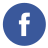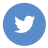### java date arithmetic

java

To perform arithmetic operations on java.time.LocalDate objects, you can use the plus() and minus() methods with a java.time.Period object. A Period object represents a specific number of days, months, and years.

Here’s an example:

``````import java.time.LocalDate;
import java.time.Period;

public class Main {
public static void main(String[] args) {
LocalDate date = LocalDate.now();
System.out.println("Current date: " + date);

// Add 1 day, 2 months, and 3 years to the current date
LocalDate future = date.plus(Period.of(3, 2, 1));
System.out.println("Future date: " + future);

// Subtract 5 days, 4 months, and 2 years from the current date
LocalDate past = date.minus(Period.of(2, 4, 5));
System.out.println("Past date: " + past);
}
}``````

You can also use the plusDays(), plusMonths(), and plusYears() methods to add or subtract specific numbers of days, months, or years:

``````import java.time.LocalDate;

public class Main {
public static void main(String[] args) {
LocalDate date = LocalDate.now();
System.out.println("Current date: " + date);

// Add 100 days to the current date
LocalDate future = date.plusDays(100);
System.out.println("Future date: " + future);

// Subtract 1 month and 3 years from the current date
LocalDate past = date.minusMonths(1).minusYears(3);
System.out.println("Past date: " + past);
}
}``````